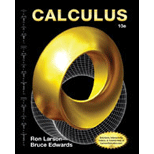# The capitalized cost C of an asset for n = 5 years when the original investment is C 0 = $650 , 000 , the annual interest rate compounded continuously is r = 0.06 and the annual cost of maintenance is C ( t ) =$ 25 , 000 ( 1 + 0.08 t ) .### Calculus

10th Edition
Ron Larson + 1 other
Publisher: Cengage Learning
ISBN: 9781285057095### Calculus

10th Edition
Ron Larson + 1 other
Publisher: Cengage Learning
ISBN: 9781285057095

#### Solutions

Chapter 8.8, Problem 82E

(a)

To determine

## To calculate: The capitalized cost C of an asset for n=5 years when the original investment is C0=$650,000, the annual interest rate compounded continuously is r=0.06 and the annual cost of maintenance is C(t)=$25,000(1+0.08t).

(b)

To determine

(c)

To determine

### Want to see the full answer?

Check out a sample textbook solution.

### Want to see this answer and more?

Experts are waiting 24/7 to provide step-by-step solutions in as fast as 30 minutes!*

*Response times may vary by subject and question complexity. Median response time is 34 minutes for paid subscribers and may be longer for promotional offers.